The MODECLUS Procedure

Computational Resources

The MODECLUS procedure stores coordinate data in memory if there is enough space. For distance data, only one observation at a time is in memory.

PROC MODECLUS constructs lists of the neighbors of each observation. The total space required is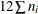bytes, where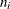is based on the largest neighborhood required by any analysis. The lists are stored in a SAS utility data set unless you specify the CORE option. You might get an error message from the SAS System or from the operating system if there is not enough disk space for the utility data set. Clustering method 6 requires a second list that is always stored in memory.

For coordinate data, the time required to construct the neighbor lists is roughly proportional to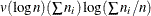. For distance data, the time is roughly proportional to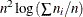.

The time required for density estimation is proportional to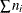and is usually small compared to the time required for constructing the neighbor lists.

Clustering methods 0 through 3 are quite efficient, requiring time proportional to. Methods 4 and 5 are slower, requiring time roughly proportional to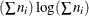. Method 6 can also be slow, but the time requirements depend very much on the data and the particular options specified. Methods 4, 5, and 6 also require more memory than the other methods.

The time required for significance tests is roughly proportional to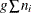, where g is the number of clusters.

PROC MODECLUS can process data sets of several thousand observations if you specify reasonable smoothing parameters. Very small smoothing values produce many clusters, whereas very large values produce many neighbors; either case can require excessive time or space.